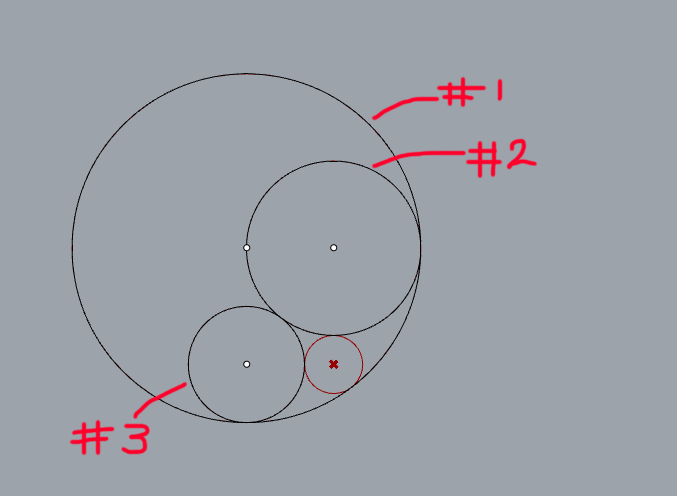# Problems with basic Apollonian Circle Packing

Good afternoon, I might be a bit a square because I can’t seem to get the basics of Apollonian circle packing.

I’ve used both the definition of curvature and Complex Descartes Theorem to draw the newest circle, and it works great in the first iteration, but when I repeat this for the second iteration it somehow fails the calculation by placing the newest circle in the wrong area and the wrong size. (refer to images ⍗)

Iteration #1Iteration #2My question is where have I gone wrong? is there something I’m not understanding about these equations, or coded something incorrectly?

I’ve included the program I put together using python if that’s any help, but this is also included in the attached files.

``````import cmath

k1=float(K1)
k2=float(K2)
k3=float(K3)

z1=complex(X1,Y1)
z2=complex(X2,Y2)
z3=complex(X3,Y3)